# Lab 3Jan 23 2019

library(tidyverse)
library(broom)
library(gganimate)

## Learning Objectives

• Use simulation to demonstrate the properties of the least squares estimates
• Explore the relationship between the covariance of the least squares estimates and the design matrix

## General idea

In this lab you’ll explore some of the properties of the least squares estimates that we have discussed in class through simulation.

Our general approach will be to:

• Fix the sample size $$n$$, the design matrix $$X$$ and a model (values for $$\beta$$ and $$\sigma^2$$) i.e. fix a simulation setting

• Repeat many times:

• simulate data by simulating the errors then generating the response according to the model
• fit the regression model and extract the parameter estimates
• Examine the parameter estimates over the many simulated datasets

In this lab we’ll focus on an ideal setting, that is, our errors will be i.i.d Normal and the model we fit will have the same form as the true model.

## Simulating one example

n <- 30

Let’s walk through the simulation once before doing it many times. Let’s consider an experiment where we have 30 seedlings, which we randomly assign to receive either 2, 7, 12, 17 or 22 hours of artificial sunlight in a day. After four weeks, the plants are harvested, dried and weighed (in grams) to give a biomass yield response.

(If you are working in the Rmarkdown document, you’ll see I defined n in a code chunk and then referenced it in the text with  r n. This is one way to allow changes to the simulation set up that get directly reflected in the narrative.)

growth <- tibble(
sunlight = rep(seq(2, 22, by = 5), length = n)
)

We’ll imagine that this simple linear regresssion model is appropriate in this setting: $\text{yield}_i = \beta_0 + \beta_1\text{sunlight}_i + \epsilon_i \quad i = 1, \ldots, 30$ where $$\epsilon_i \sim_{\text{i.i.d}} N(0, \sigma^2)$$.

beta_0 <- 5
beta_1 <- 0.5
sigma_sq <- 4

For a simulation we’ll need to set values for $$\beta_0$$, $$\beta_1$$ and $$\sigma^2$$, let’s say $\beta_0 = 5, \quad \beta_1 = 0.5, \quad \sigma^2 = 4$

(You might argue if these are realistic…you are also welcome to suggest a better example).

We can then simulate a single example of the experiment outcome:

sim_1 <- growth %>%
mutate(
error = rnorm(n, mean = 0, sd = sqrt(sigma_sq)),
yield = beta_0 + beta_1 * sunlight + error
)

And take a look at the simulated data:

ggplot(sim_1, aes(sunlight, yield)) +
geom_point() +
geom_smooth(method = "lm", se = FALSE)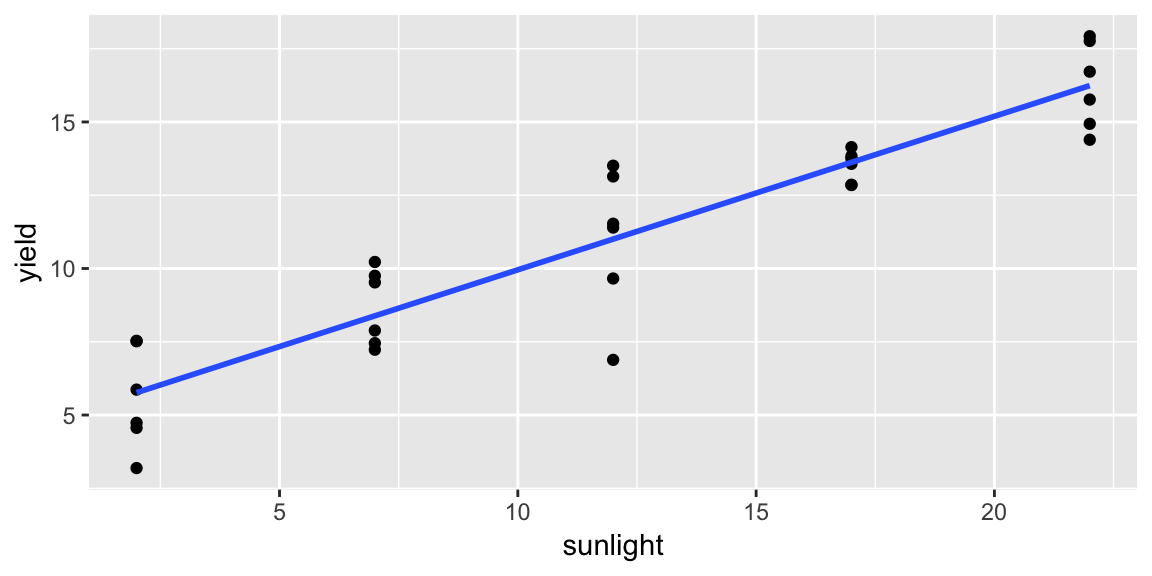We can fit a model to our data:

fit_lm <- lm(yield ~ sunlight, data = sim_1)

And look at the result:

summary(fit_lm)

Our estimates obviously don’t match the truth, the question is: over many such experiments how do our estimates behave? We’ve derived the answer in class, but I think it helps to see it through simulation too.

It will be useful to use the broom::tidy() function which takes a model and produces a tidy tibble of the regression coefficient estiamtes

tidy(fit_lm)
##          term  estimate std.error statistic      p.value
## 1 (Intercept) 4.7179088 0.5548150  8.503571 3.030119e-09
## 2    sunlight 0.5237286 0.0398334 13.147976 1.675672e-13

We’ll just need the estimates and their labels which we can grab with dplyr::select()

tidy(fit_lm) %>%
select(term, estimate)
##          term  estimate
## 1 (Intercept) 4.7179088
## 2    sunlight 0.5237286

## Simulating many times

There are lot’s of different ways (code-wise) we could repeat this process many times. I tend to like the tidyverse style of using list columns in combination with purrr:map() and dplyr::mutate(), maybe you prefer for loops. I’ve got some code below (in the Rmarkdown) to repeat our simulation many times, but it’s not too important to understand all the steps.

It’s more important to me that you understand the process of the simulation. To help you visualize what is happening I’ve constructed this animation: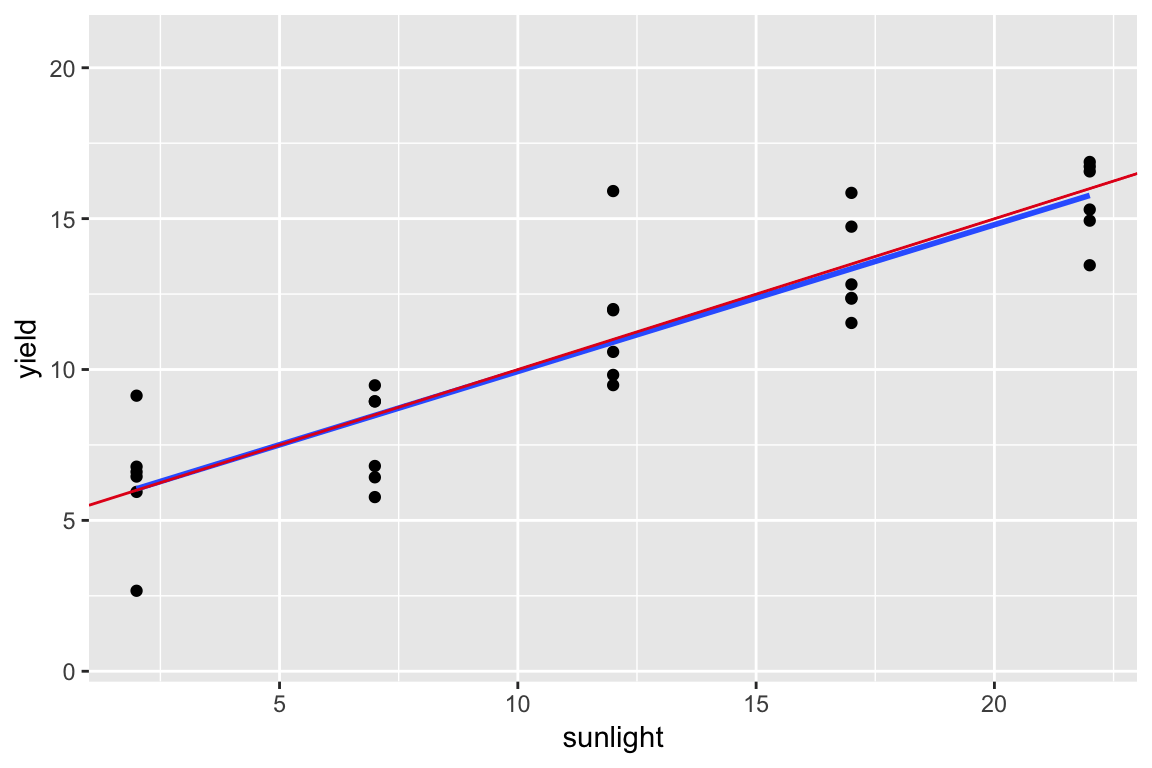Each frame has one simulated data set on it (the black points), and the resulting model fit (the blue line). I’ve added the true model as the red line, and previous fits remain as pale grey lines. Your should see that although any individual line may not be that close to the true line, but on average, they tend to be good (i.e. the fitted lines are unbiased).

In my simulation, the important piece of output is sim_coefs:

sim_coefs
## # A tibble: 200 x 3
##      sim term        estimate
##    <int> <chr>          <dbl>
##  1     1 (Intercept)    5.07
##  2     1 sunlight       0.486
##  3     2 (Intercept)    6.40
##  4     2 sunlight       0.421
##  5     3 (Intercept)    6.65
##  6     3 sunlight       0.400
##  7     4 (Intercept)    4.99
##  8     4 sunlight       0.505
##  9     5 (Intercept)    5.31
## 10     5 sunlight       0.526
## # ... with 190 more rows

It has a row for each coefficient estimate, where sim indentifies which simulation the estimate came from. So for example, the estimates from the first simulation are:

sim_coefs %>%
filter(sim == 1)
## # A tibble: 2 x 3
##     sim term        estimate
##   <int> <chr>          <dbl>
## 1     1 (Intercept)    5.07
## 2     1 sunlight       0.486

We can investigate the parameters individually by looking at histograms of the estimates:

ggplot(sim_coefs, aes(estimate)) +
geom_histogram() +
facet_wrap(~ term, scale = "free_x")
## stat_bin() using bins = 30. Pick better value with binwidth.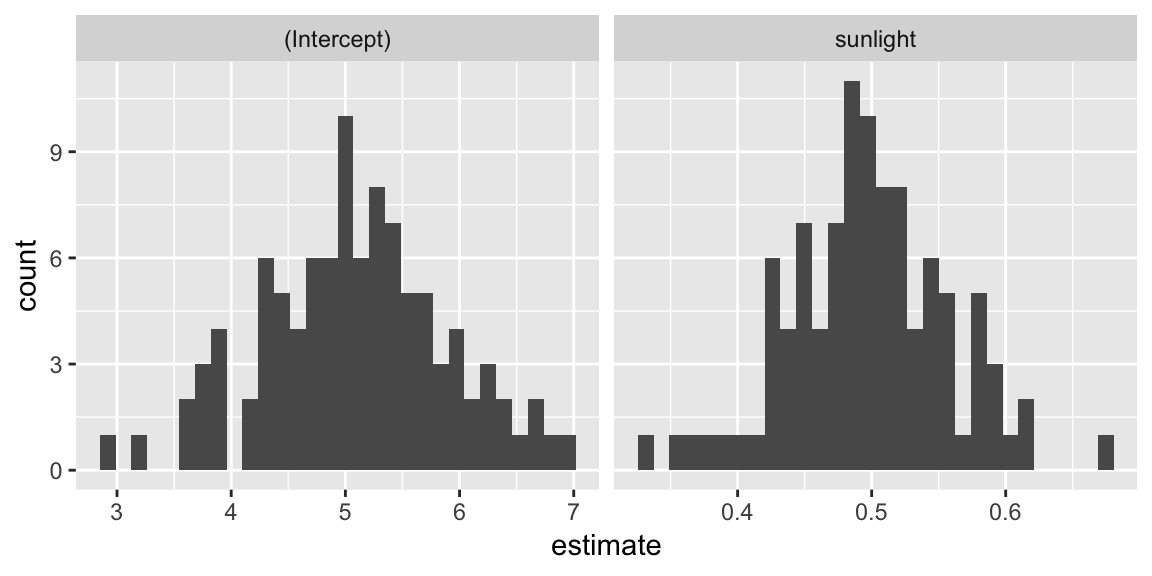Your turn What does the theory say these should look like? Do they look reasonable?

We can also look at the relationship between the esitmate for the slope and intercept by plotting them on a scatterplot:

sim_coefs %>%
ggplot(aes((Intercept), sunlight)) +
geom_point()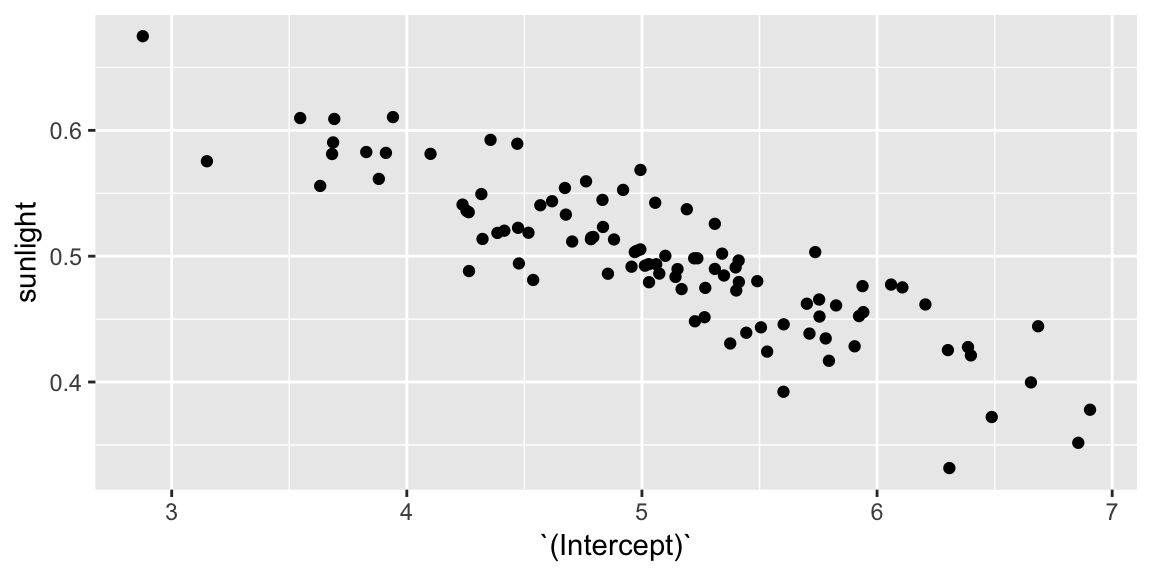Your turn How would describe the relationship between the two estimates? Go back to the animation, can you give intuition as to why this happens?

Recall from class this covariance depends on $$\sigma^2$$ and $$X$$ through $\text{Var}\left(\hat{\beta}\right) = \sigma^2 \left(X^T X \right)^{-1}$

We can get the design matrix $$X$$ from the original fit in our first simulation:

X <- model.matrix(fit_lm)

• Use the formula above to calculate the true variance-covariance matrix for the estimates.

• Convert it to a correlation matrix with the cov2cor() function.

• Does it agree with your observation from the scatterplot?

## Centering

We have some control over the form of $$X$$ from how we set up our regression model. In this section, you’ll explore the effect of centering the explanatory variable of the relationship between the estimates of the slope and intercept.

Centering the explanatory variable means subtracting the mean of the explanatory model before fitting, or in other words changing our model to: $\text{yield}_i = \gamma_0 + \gamma_1\left(\text{sunlight}_i - \overline{\text{sunlight}}\right) + \epsilon_i \quad i = 1, \ldots, 30$

Visually, this is the new model:

ggplot(sim_1, aes(sunlight - mean(sunlight), yield)) +
geom_point() +
geom_smooth(method = "lm", se = FALSE)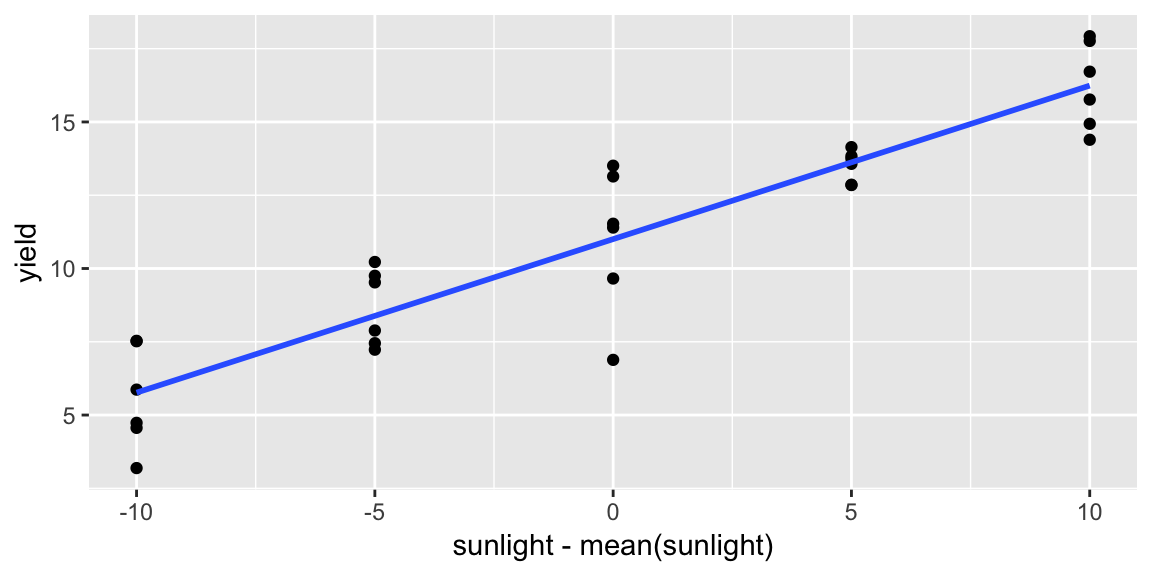Notice we’ve just shifted the x-axis, otherwise everything is identical.

We can repeat the simulation now fitting this new centered model (see Rmarkdown for code).

Resulting in an similar object with the fitted coefficients:

sim_coefs_centered
## # A tibble: 200 x 3
##      sim term                         estimate
##    <int> <chr>                           <dbl>
##  1     1 (Intercept)                    10.9
##  2     1 I(sunlight - mean(sunlight))    0.486
##  3     2 (Intercept)                    11.5
##  4     2 I(sunlight - mean(sunlight))    0.421
##  5     3 (Intercept)                    11.5
##  6     3 I(sunlight - mean(sunlight))    0.400
##  7     4 (Intercept)                    11.1
##  8     4 I(sunlight - mean(sunlight))    0.505
##  9     5 (Intercept)                    11.6
## 10     5 I(sunlight - mean(sunlight))    0.526
## # ... with 190 more rows

Your turn How do the $$\gamma$$s relate to the $$\beta$$s in the orginal model? You might verify your intuition by comparing the values in sim_coefs to sims_coefs_centered.

• Make a scatterplot of the estimated slope and intercept, how is the relationship different in this model?

• Verify your observation by deriving the correlation between the estimates from the variance-covariance matrix.

Setting up design matrices to have orthogonal columns is a large part of experimental design. It allows the the estimates of certain factors to be less dependent on which other factors are included in the model.

## Further exploration

The following code pulls out the estimate of $$\sigma$$ for each simulation:
sims_sigma_hats <- sims %>%
select(sim, sigma, r.squared)
Can you verify $$\hat{\sigma^2}$$ is an unbiased estimate of $$\sigma^2$$?